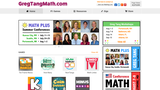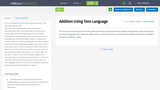# Search Results (131)

View
Selected filters:Restricted Use
Rating

This is a game where students practice adding and decomposing sums up ...

This is a game where students practice adding and decomposing sums up to 12.  The game is for 2 players and requires two six-sided dice, and a 10-Pin Limbo! game sheet.

Subject:
Mathematics
Material Type:
Game
Provider:
Creative Smarts, Inc.
01/18/2017Conditional Remix & Share Permitted
CC BY-NC-SA
Rating

This problem helps students practice adding three numbers whose sum are 20 ...

This problem helps students practice adding three numbers whose sum are 20 or less.

Subject:
Mathematics
Numbers and Operations
Material Type:
Activity/Lab
Provider:
Illustrative Mathematics
Provider Set:
Illustrative Mathematics
Author:
Illustrative Mathematics
09/10/2012Conditional Remix & Share Permitted
CC BY-NC-SA
Rating

The purpose of this task is to study some patterns in a ...

The purpose of this task is to study some patterns in a small addition table. Each pattern identified persists for a larger table and if more time is available for this activity students should be encouraged to explore these patterns in larger tables.

Subject:
Mathematics
Numbers and Operations
Material Type:
Activity/Lab
Provider:
Illustrative Mathematics
Provider Set:
Illustrative Mathematics
Author:
Illustrative Mathematics
08/15/2012Rating

Students use this puzzle to practice 2-digit addition. There are two puzzles: ...

Students use this puzzle to practice 2-digit addition. There are two puzzles: one without regrouping and one with regrouping. These puzzles make a great math station/center as students are able to independently check their work. Students can also use this resource as a math choice for when they finished their work.

Subject:
Mathematics
Material Type:
Lesson
Lesson Plan
Provider:
Rachel Rolfs
Author:
Rachel Rolfs
03/28/2018Unrestricted Use
CC BY
Rating

This is a modification of the Illustrative Math lesson, "Ford and Logan ...

This is a modification of the Illustrative Math lesson, "Ford and Logan add 45 + 36," https://www.illustrativemathematics.org/content-standards/tasks/2068. This lesson was used with a tier 3 fourth grade intervention math group to help students develop their understanding of place value and use of tens language when adding two digit numbers. I modified it to meet the students' needs by adding ways two other students (Sarah and Tammy) solved the problem: Sarah said, "I know 4 tens plus 3 tens is 7 tens; 5 ones plus 6 ones is eleven ones which is 1 ten and 1 one so 4 tens plus 3 tens plus 1 tens is 8 tens and the one left so the answer is 81." Tammy said, " 45 plus 30 is 75, plus 6 more is 81." Students analyzed and discussed all four methods and compared them to their own methods. I then had students practice using the tens and ones language (like Sarah) for some more addition problems without using place value blocks or models.

Subject:
Mathematics
Material Type:
Author:
MEGAN HINDE
08/02/2019Rating

In this data analysis activity students investigate data in connection with recyclable ...

In this data analysis activity students investigate data in connection with recyclable materials and develop plans to help the environment. Students collect data about aluminum can usage and graph that data in a line plot. The lesson includes student worksheet and extension suggestions.

Subject:
Mathematics
Material Type:
Interactive
Lesson Plan
Provider:
National Council of Teachers of Mathematics
Provider Set:
Illuminations
11/05/2008Conditional Remix & Share Permitted
CC BY-NC-SA
Rating

These 3 word problems require students to solve addition and subtraction problems.

Subject:
Mathematics
Numbers and Operations
Material Type:
Activity/Lab
Provider:
Illustrative Mathematics
Provider Set:
Illustrative Mathematics
Author:
Illustrative Mathematics
05/01/2012Rating

In this lesson students use a rule of thumb about the weight ...

In this lesson students use a rule of thumb about the weight of babies to practice doubling and halving numbers. They complete an organized table and compare data using vertical and horizontal double bar graphs. The lesson includes a student activity sheet and extension ideas.

Subject:
Mathematics
Material Type:
Interactive
Lesson Plan
Provider:
National Council of Teachers of Mathematics
Provider Set:
Illuminations
Author:
Marcy Cook
03/31/2008Rating

In this lesson plan students conduct a survey of ten students, collect ...

In this lesson plan students conduct a survey of ten students, collect data in a tally chart, and then display and analyze the results in a bar graph. In the analysis students must compare numbers, add, and subtract using the data in the bar graph to generate questions as well as answer them. The lesson plan includes a graph template, a link to an interactive graphing tool, extension questions, and a fact family sheet to show the related facts from their graph analysis.

Subject:
Mathematics
Material Type:
Interactive
Lesson Plan
Provider:
National Council of Teachers of Mathematics
Provider Set:
Illuminations
Author:
Grace M. Burton
11/05/2008Rating

In this 8-lesson unit students use buttons to explore logical and numerical ...

In this 8-lesson unit students use buttons to explore logical and numerical relationships that form the conceptual basis for understanding addition and subtraction operations. Topics include counting, ordinal numbers (and relative position), classification (attributes), relationships between numbers, addition of sets, commutativity of addition, sums to 10, fact families (including subtraction), three models of subtraction ("take away", comparative, missing addend), and bar graphs. Includes student activity sheets and a link to an online graphing applet.

Subject:
Mathematics
Material Type:
Interactive
Lesson Plan
Provider:
National Council of Teachers of Mathematics
Provider Set:
Illuminations
Author:
Grace M. Burton
11/05/2000Rating

In this 8-lesson unit students use buttons to explore logical and numerical ...

In this 8-lesson unit students use buttons to explore logical and numerical relationships that form the conceptual basis for understanding addition and subtraction operations. Topics include counting, ordinal numbers (and relative position), classification (attributes), relationships between numbers, addition of sets, commutativity of addition, sums to 10, fact families (including subtraction), three models of subtraction ("take away", comparative, missing addend), and bar graphs. Includes student activity sheets and a link to an online graphing applet.

Subject:
Mathematics
Material Type:
Interactive
Lesson Plan
Provider:
National Council of Teachers of Mathematics
Provider Set:
Illuminations
Author:
Grace M. Burton
03/31/2000Rating

In this Illuminations lesson plan students explore the use of variables as ...

In this Illuminations lesson plan students explore the use of variables as they solve for the weights of objects using information presented in pictures. Learners model situations that involve adding and subtracting whole numbers, using objects, pictures, and symbols. A student activity sheet is available and downloadable (pdf).

Subject:
Mathematics
Algebra
Material Type:
Interactive
Lesson Plan
Provider:
National Council of Teachers of Mathematics
Provider Set:
Illuminations
11/05/2009Conditional Remix & Share Permitted
CC BY-NC-SA
Rating

The purpose of this task is for students to find different pairs ...

The purpose of this task is for students to find different pairs of numbers that sum to 4.

Subject:
Mathematics
Measurement and Data
Material Type:
Activity/Lab
Provider:
Illustrative Mathematics
Provider Set:
Illustrative Mathematics
Author:
Illustrative Mathematics
05/01/2012Conditional Remix & Share Permitted
CC BY-NC-SA
Rating

Students use addition or subtraction to solve these types of word problems.

Subject:
Mathematics
Numbers and Operations
Material Type:
Activity/Lab
Provider:
Illustrative Mathematics
Provider Set:
Illustrative Mathematics
Author:
Illustrative Mathematics
05/01/2012Rating

In this lesson, students explore arithmetic patterns to help solve a real-world ...

In this lesson, students explore arithmetic patterns to help solve a real-world problem involving addition operations. This lesson supports the 3rd grade standard of identifying arithmetic patterns and explaining them using properties of operations.

Subject:
Mathematics
Material Type:
Lesson Plan
Provider:
Dan Meyer
12/28/2015Conditional Remix & Share Permitted
CC BY-NC-SA
Rating

This lesson integrates language arts, music, and math. The children will listen ...

This lesson integrates language arts, music, and math. The children will listen to the story "Count on Bunnies". They will be given the opportunity to act out the story and solve bunny equations. After listening to the song "Five Young Rabbits," the children will take turns being rabbits and pantomiming the actions as the class sings. The children will combine the rabbits at the end of each verse to see how many rabbits have been added. Then they will work in pairs to create their own rabbit equations.

Subject:
Fine Arts
Numbers and Operations
Material Type:
Lesson Plan
Provider:
University of North Carolina at Chapel Hill School of Education
Provider Set:
LEARN NC Lesson Plans
Author:
Becky Smith
06/25/1999Only Sharing Permitted
CC BY-NC-ND
Rating

I used The Hungry Catepillar lesson from this link. https://www.illustrativemathematics.org/content-standards/tasks/1150 However I ...

I used The Hungry Catepillar lesson from this link.

However I did an extension off this lesson using this resource to allow teachers to work on the skills of addition, the precise language of tens and some extra ones, and seeing groups of ten.

This activity is a great way to include Language Arts along with Mathematics. Using The Very Hungry Catepillar, we used based-ten blocks to place onto a ten frames. Each time the catepillar eats something, we add to the ten frame. At the end of the story, we look at how much the catepillar has eaten. However, I took this activity a step further to continue strengthening the use of addition. Students and I then had a variety of situations at the end of the story where the catepillar ate 4 more leaves. The students then add four more, either in a group of 4 or counting out 4 individual items. We then look again at using our language of, "I have a 3 groups of ten and 4 extra ones."

Challenges with this lesson is making sure the students have added a cube onto their ten frame for each item they ate. As well, students would have to also understand how to use a ten frame before the start of the lesson. As well, if you are working to be precise with the language of tens and extra ones, you want to nudge your students to use this language. You will want to model this multiple times.

Subject:
Mathematics
Material Type:
Author:
Alicia Korth
08/02/2019Rating

In this lesson from Illuminations, students explore and discover linear relationships. Linear ...

In this lesson from Illuminations, students explore and discover linear relationships. Linear patterns are identified, extended and described verbally, numerically and algebraically through three investigations. Using manipulatives and the linked applet, "Chairs", learners determine the number of chairs needed when the number of tables is known, and vice versa. Instructional plan, questions for the students, assessment options, extensions and teacher reflections are provided.

Subject:
Mathematics
Algebra
Functions
Material Type:
Interactive
Lesson Plan
Provider:
National Council of Teachers of Mathematics
Provider Set:
Illuminations
Author:
Samuel E. Zordak
11/05/2011Conditional Remix & Share Permitted
CC BY-NC-SA
Rating

The purpose of this task is for students to find different pairs ...

The purpose of this task is for students to find different pairs of numbers that sum to 7.

Subject:
Mathematics
Measurement and Data
Material Type:
Activity/Lab
Provider:
Illustrative Mathematics
Provider Set:
Illustrative Mathematics
Author:
Illustrative Mathematics